ControlNet 和 Stable Diffusion 的结合，可以让SD接受条件输入，以引导图像生成过程，得到增强版的 SD.

Github - lllyasviel/ControlNet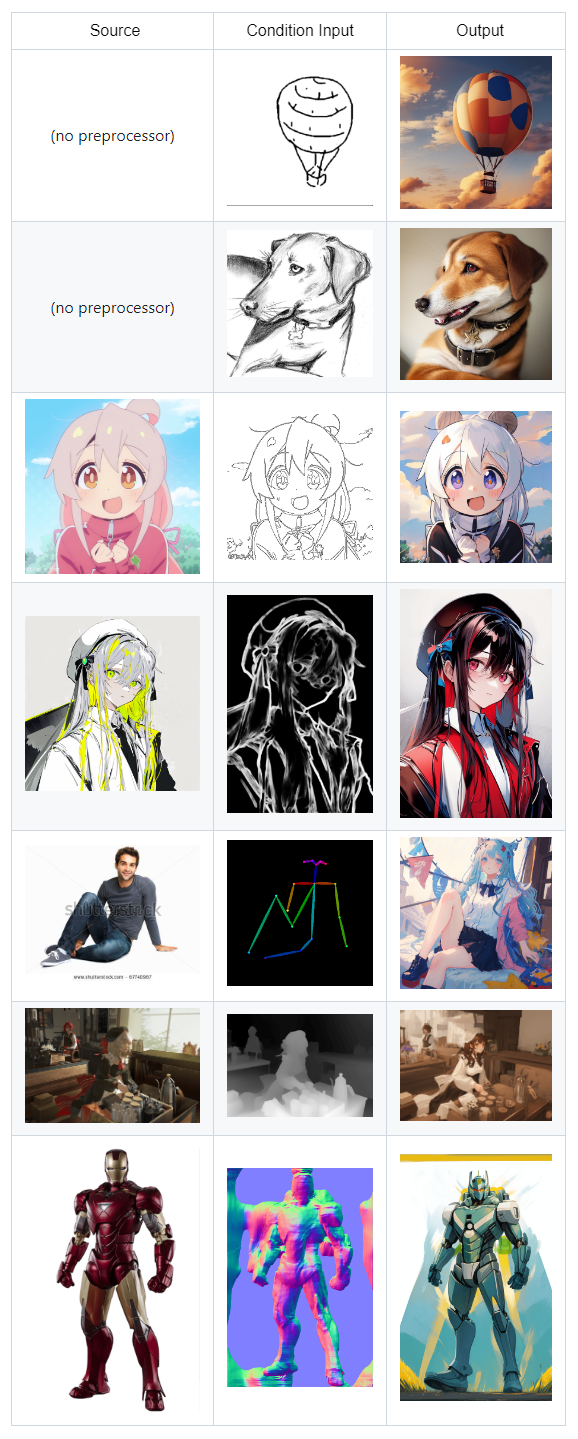## ControlNet 结构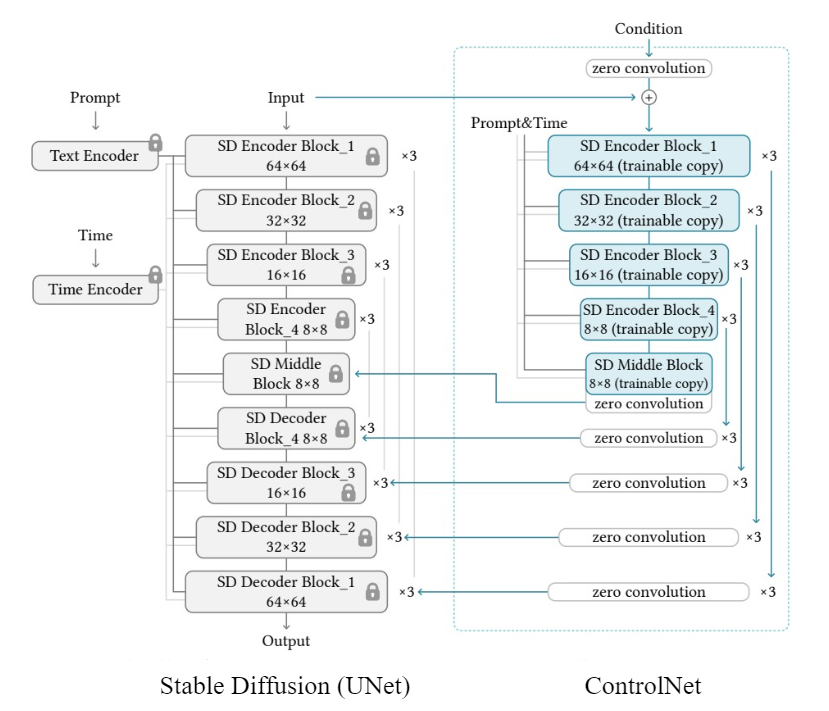SD UNet 中的所有参数被冻结，并克隆一份(trainable copy)到 ControlNet. 这些 trainable copy 通过一个外部条件向量(external condition vector)进行训练.

• 避免数据集比较小时的过拟合
• 保持大模型的高质量性能

### Feedforward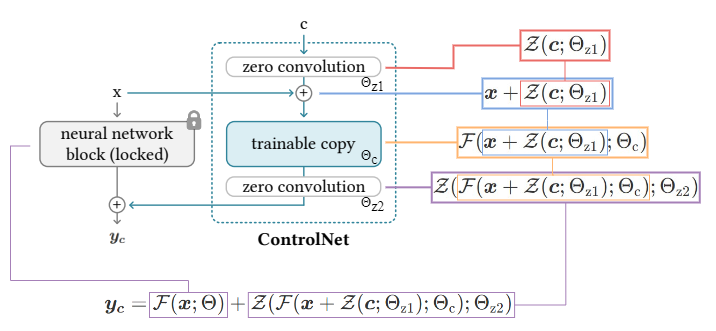- $x, y$ - 神经网络中的深度特征

 - $c$ - 额外的条件

 - $+$ - 特征相加，Feature addition

 - $Z(\cdot;\cdot)$ - Zero convolution 操作，weight 和 bias 初始化都是 0 的 1x1 conv 层

 - $F(\cdot;\cdot)$ - 神经网络 block 操作，如 resnet block，conv-bn-relu block 等

 - $\Theta _{z1}$ - 第一个 zero conv 层的参数

 - $\Theta _{z2}$ - 第二个 zero conv 层的参数

 - $\Theta _{c}$ - 训练副本(trainable copy) 的参数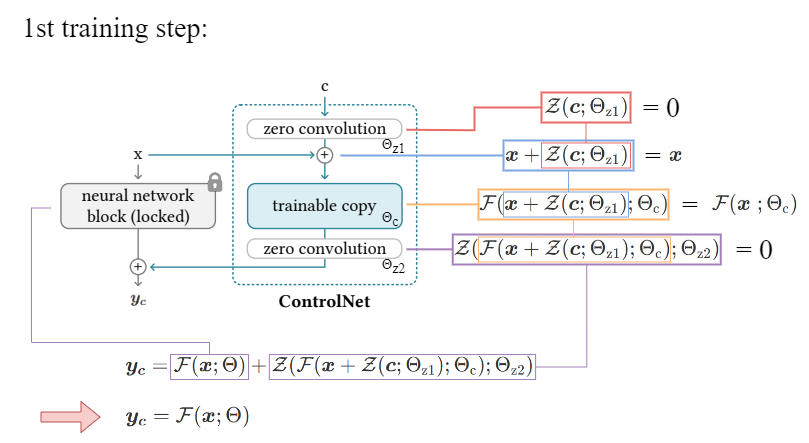### Backpropagation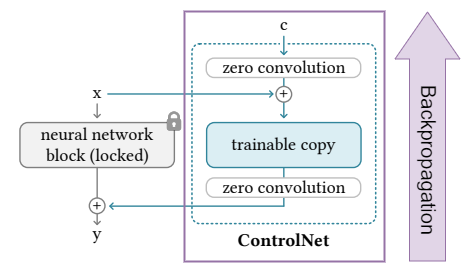backpropagation 更新 trainable copy 和 ControlNet 中的 zero conv 层的参数，使得 zero conv 的权重通过学习过程逐渐过渡到优化值.

#### Why gradient will not be zero?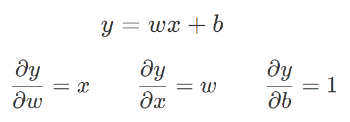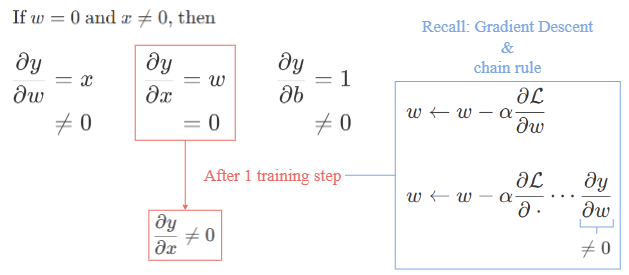## ControlNet with Stable Diffusion

### Encoder

$$c_f = \xi (c_i)$$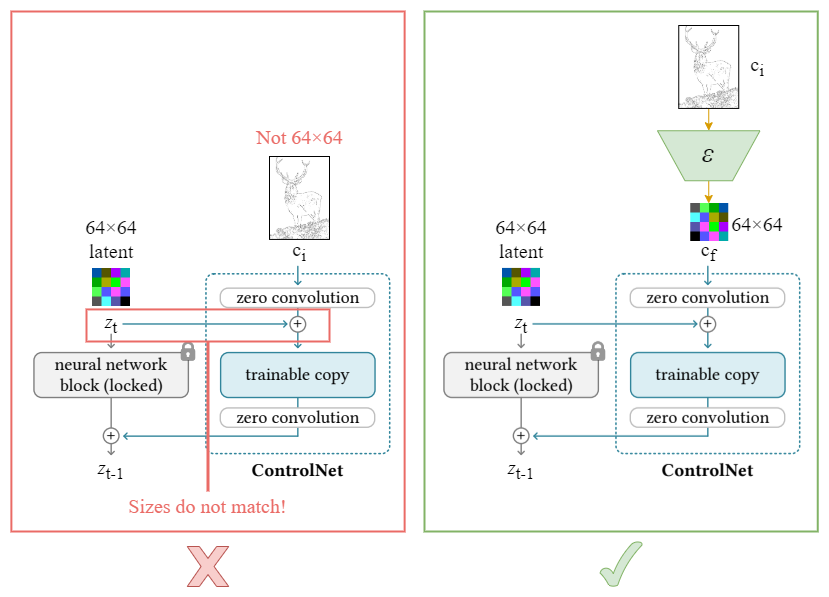### Overall Architecture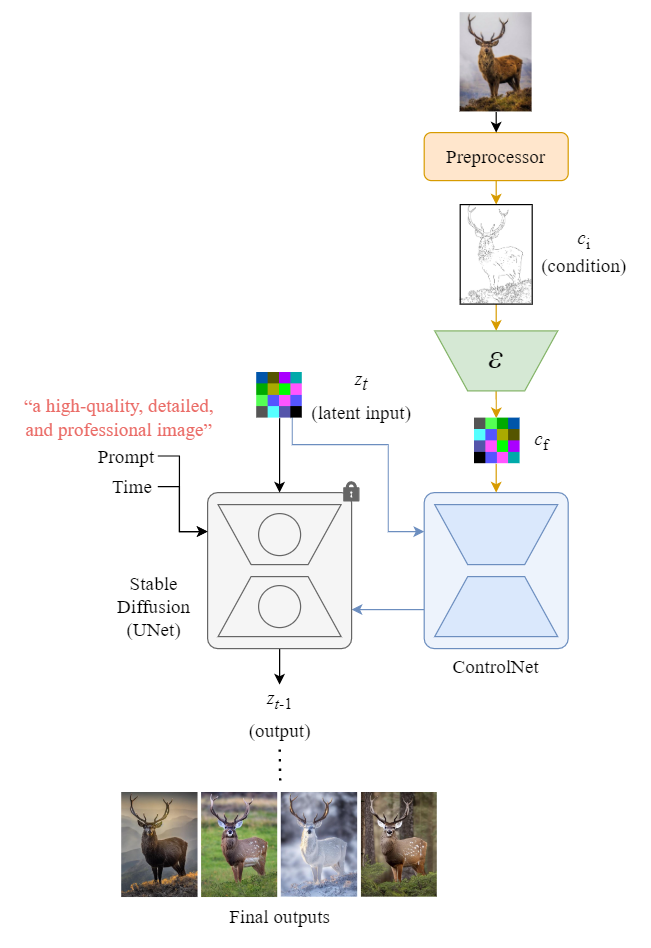ControlNet 和 SD 的 reverse diffusion process (sampling) 示例，如图：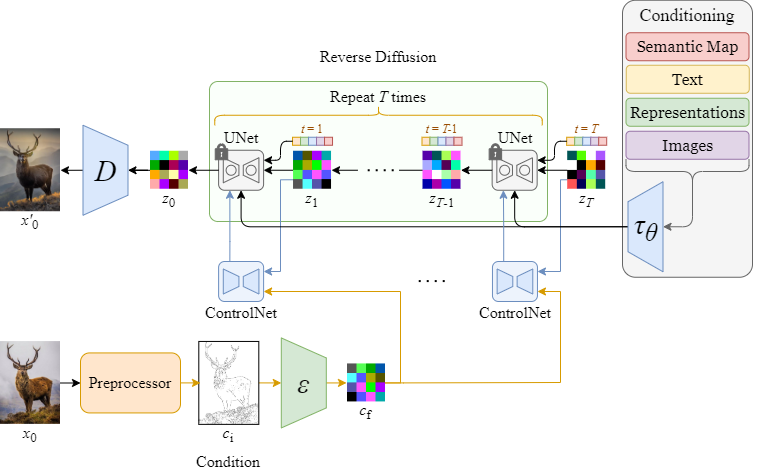### Training

ControlNet 的损失函数类似于 SD，但包含了 text condition $c_t$ 和 latent condition $c_f$，以基于特定条件提升输出一致性.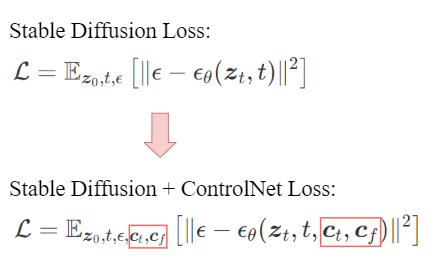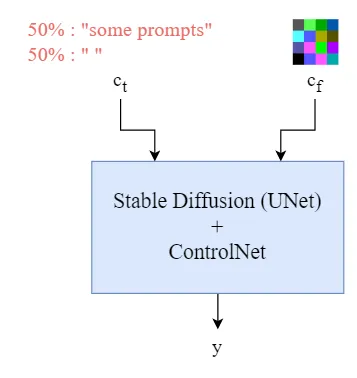## Summary

ControlNet 是与预训练的 Diffusion Model，特指 Stable Diffusion，共同使用的一种神经网络。

ControlNet 允许包含更多的条件输入，如edge maps, segmentation maps, and key points 等.

## References

 L. Zhang and M. Agrawala, “Adding conditional control to text-to-image diffusion models,” arXiv.org, https://arxiv.org/abs/2302.05543.

Last modification：June 13th, 2023 at 04:59 pm